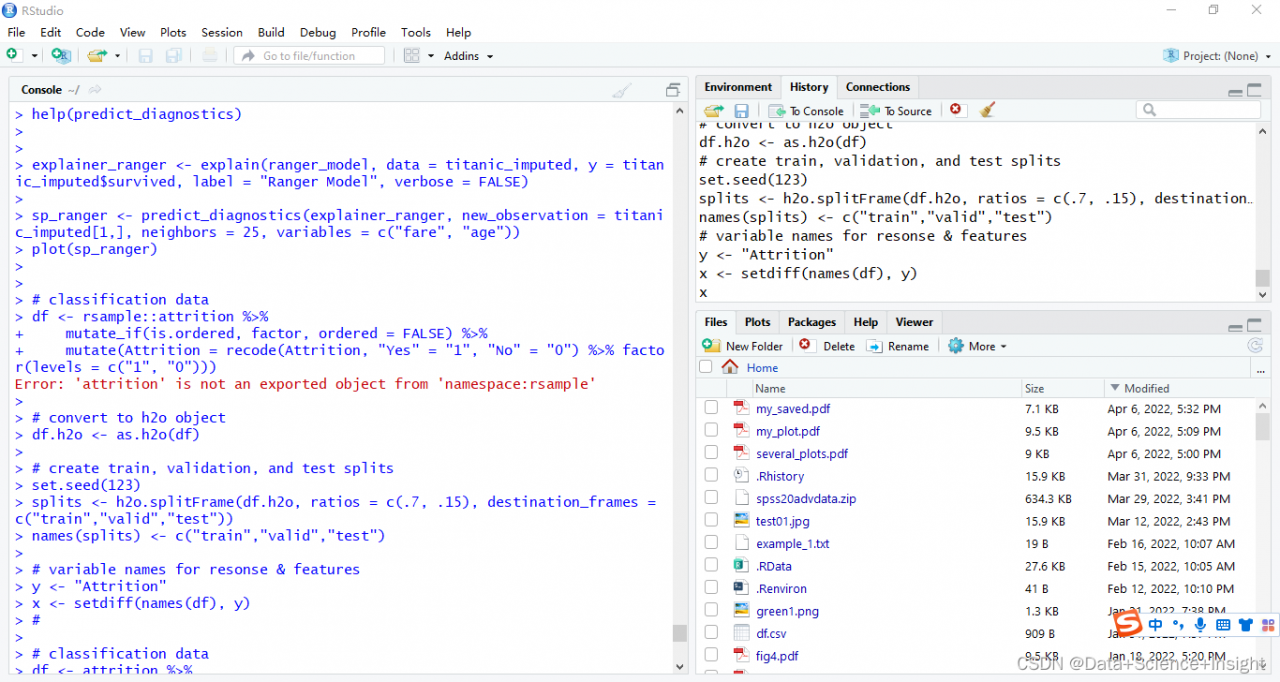# [Solved] Error: ‘attrition‘ is not an exported object from ‘namespace:rsample‘

Error: ‘attrition’ is not an exported object from ‘namespace:rsample’

# Import package and library

``````# load required packages
library(rsample)
library(dplyr)
library(h2o)
library(DALEX)

# initialize h2o session
h2o.no_progress()
h2o.init()
##  Connection successful!
##
## R is connected to the H2O cluster:
##     H2O cluster uptime:         4 hours 30 minutes
##     H2O cluster timezone:       America/New_York
##     H2O data parsing timezone:  UTC
##     H2O cluster version:        3.18.0.11
##     H2O cluster version age:    1 month and 17 days
##     H2O cluster total nodes:    1
##     H2O cluster total memory:   1.01 GB
##     H2O cluster total cores:    4
##     H2O cluster allowed cores:  4
##     H2O cluster healthy:        TRUE
##     H2O Connection ip:          localhost
##     H2O Connection port:        54321
##     H2O Connection proxy:       NA
##     H2O Internal Security:      FALSE
##     H2O API Extensions:         XGBoost, Algos, AutoML, Core V3, Core V4
##     R Version:                  R version 3.5.0 (2018-04-23)``````

#Data preprocessing and processing to h2o format;

Error: ‘attrition’ is not an exported object from ‘namespace:rsample’

``````#

# classification data
df <- rsample::attrition %>%
mutate_if(is.ordered, factor, ordered = FALSE) %>%
mutate(Attrition = recode(Attrition, "Yes" = "1", "No" = "0") %>% factor(levels = c("1", "0")))

# convert to h2o object
df.h2o <- as.h2o(df)

# create train, validation, and test splits
set.seed(123)
splits <- h2o.splitFrame(df.h2o, ratios = c(.7, .15), destination_frames = c("train","valid","test"))
names(splits) <- c("train","valid","test")

# variable names for resonse & features
y <- "Attrition"
x <- setdiff(names(df), y) ``````

Solution:

You can use the attrition dataset of DALEX package directly;

Remove resample:

``````#

# classification data
df <- attrition %>%
mutate_if(is.ordered, factor, ordered = FALSE) %>%
mutate(Attrition = recode(Attrition, "Yes" = "1", "No" = "0") %>% factor(levels = c("1", "0")))

# convert to h2o object
df.h2o <- as.h2o(df)

# create train, validation, and test splits
set.seed(123)
splits <- h2o.splitFrame(df.h2o, ratios = c(.7, .15), destination_frames = c("train","valid","test"))
names(splits) <- c("train","valid","test")

# variable names for resonse & features
y <- "Attrition"
x <- setdiff(names(df), y) ``````

Full Error Messages:

```> # classification data
> df <- rsample::attrition %>%
+     mutate_if(is.ordered, factor, ordered = FALSE) %>%
+     mutate(Attrition = recode(Attrition, "Yes" = "1", "No" = "0") %>% factor(levels = c("1", "0")))
Error: 'attrition' is not an exported object from 'namespace:rsample'
>```